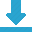# A Simple Tool To Convert Between Total Growth And Annualised Growth Rate

1/7/2019.  This simple spreadsheet lets you quickly and accurately convert between compound annual growth rate (CAGR) and total growth, in either percentages or numerical terms.

You can easily:
• Find annualised growth rate and total growth, as percentages, by entering start and end values.

• Find end value and annualised growth rate, by entering start value and total growth percentage.

• Find end value and total growth percentage, by entering start value and annualised growth rate.

Total growth to annualised growth rate converter - Download